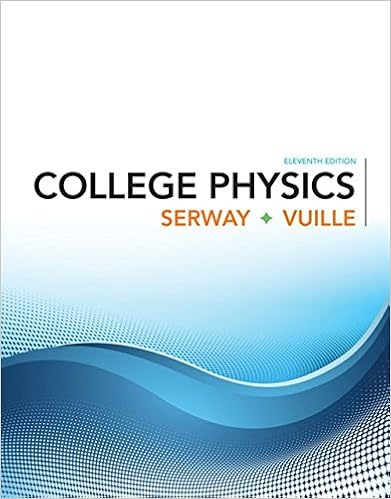# 2054_Fall15_Exam2 - 77777 77777 Instructor Field/Korytov...

• Test Prep
• 13
• 100% (1) 1 out of 1 people found this document helpful

This preview shows page 1 - 3 out of 13 pages.

##### We have textbook solutions for you!
The document you are viewing contains questions related to this textbook.The document you are viewing contains questions related to this textbook.
Chapter 20 / Exercise 20.1
College Physics
Serway/VuilleExpert Verified
77777 77777 Instructor: Field/Korytov PHYSICS DEPARTMENT PHY 2054 Exam 2 November 3, 2015 Name (PRINT, last, first): Signature: On my honor, I have neither given nor received unauthorized aid on this examination. YOUR TEST NUMBER IS THE 5-DIGIT NUMBER AT THE TOP OF EACH PAGE. DIRECTIONS (1) Code your test number on your answer sheet (use 76–80 for the 5-digit number) . Code your name on your answer sheet. DARKEN CIRCLES COMPLETELY . Code your student number on your answer sheet. (2) Print your name on this sheet and sign it also. (3) Do all scratch work anywhere on this exam that you like. At the end of the test, this exam printout is to be turned in. No credit will be given without both answer sheet and printout with scratch work most questions demand. (4) Blacken the circle of your intended answer completely, using a #2 pencil or blue or black ink . Do not make any stray marks or the answer sheet may not read properly. (5) The answers are rounded off. Choose the closest to exact. There is no penalty for guessing. >>>>>>>> WHEN YOU FINISH <<<<<<<< Hand in the answer sheet separately. Constants ǫ 0 = 8 . 85 × 10 12 F/m m e = 9 . 11 × 10 31 kg m p = m n = 1 . 67 × 10 27 kg e = 1 . 6 × 10 19 C k = 8 . 99 × 10 9 N m 2 /C 2 µ 0 = 12 . 56 × 10 7 H/m N A = 6 . 02 × 10 23 atoms/mole c = 3 × 10 8 m/s milli = 10 3 micro = 10 6 nano = 10 9 pico = 10 12
##### We have textbook solutions for you!
The document you are viewing contains questions related to this textbook.The document you are viewing contains questions related to this textbook.
Chapter 20 / Exercise 20.1
College Physics
Serway/VuilleExpert Verified
77777 77777 PHY2054 Exam 1 Formula Sheet Vectors z b y b x b b z a y a x a a z y x z y x ˆ ˆ ˆ ˆ ˆ ˆ + + = + + = r r Magnitudes: 2 2 2 2 2 2 z y x z y x b b b b a a a a + + = + + = r r Scalar Product: z z y y x x b a b a b a b a + + = r r θ cos b a r r = ( θ = angle between a r and b r ) Electrostatic Force and Electric Field Electrostatic Force (vector): r r q q k F ˆ 2 2 1 = r (r = distance between charge q 1 and charge q 2 , units = N) k = 1/(4 πε 0 ) = 8.99x10 9 N∙m 2 /C 2 ε 0 = 8.85x10 -12 C 2 /(N∙m 2 ) Electric Field (at q 2 due to q 1 ): r r q k q F E ˆ / 2 1 2 = = r r ( units = N/C = V/m) Electric Flux (through the infinitesimal surface area dA) : A d E d E r r = Φ (units = Nm 2 /C) Vector Area (directed area): n A A ˆ = r (where n ˆ = normal to the surface) Gauss’ Law (net flux through closed surface S ) : 0 ε enclosed S E Q A d E = = Φ r r (Q enclosed = charge enclosed) Gauss’ Law: Net flux through closed surface S = the charge enclosed by surface S divided by ε 0 Electric Potential and Potential Energy Electric Potential Energy: work done against a constant field E in moving charge q a distance d along straight line path from A to B, d E q U U U A B r r - = - = Δ (units = J) Electric Potential: Work done per unit charge against a constant field E in moving charge q a distance d along straight line path from A to B, d E q U V r r - = Δ = Δ / (units = J/C = V) Electric Potential (distance r from a point charge q) : r q k r V = ) ( N point charges: = = N i i i r q k r V 1 ) ( Electric Potential Energy (N point charges): = = N i i i V q U 1 2 1 , Stored Electric Potential Energy (N conductors with charge Q i and electric potential V i ) : = = N i i i V Q U 1 2 1 Capacitance (definition) : C = Q/V or C = Q/ Δ V (units = C/V = F) Energy Density of the Electric Field: 2 0 2 1 E u ε = (units = J/m 3 ) Electric Current and Circuits Current (through directed area A) : A v nq A
•••